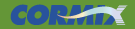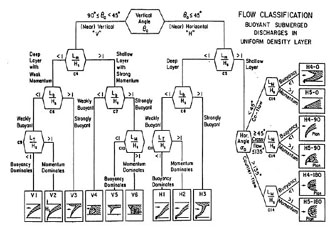# Length Scales and Mixing Processes

Within CORMIX, length scales are used for flow classification and to describe flow stability and boundary interaction for point source discharges. The flow classification describes the general flow behavior, allowing the construction of an appropriate series of regional flow models to simulate the entire near-field and far-field flow field. Length scales are also used in control volume techniques to simulate complex flows where more detailed methods are not available.

For example, on dimensional grounds the following length scales can be derived from discharge flux quantities:

• LM = M03/4 / J01/2 : This is the jet to plume transition scale, defining where flow properties change from jet-like motions to plume-like motions.
• Lm= M01/2 / ua: This is the jet to crossflow transition scale, defining where flow properties change from weakly deflected jet-like motions to strongly deflected jet-like motions.

These scales are illustrated by the images above. Length scales are not meant to be precise measurements of flow distances, only scale estimates.

##### Length Scales and CORMIX Flow Classification

Length scales describe the relative importance of discharge volume flux, momentum flux, buoyancy flux, ambient crossflow, and density stratification in controlling flow behavior. The length scales will describe the distance over which these dynamic quantities control the flow. Along with the layer height Hs, these scales can describe flow boundary interaction processes and flow stability in the near-field.

##### Basic Discharge Flux Quantities

The basic discharge flux quantities used in formulation of these length scales are:

• Discharge Volume flux: Q0 = u0A0
• Discharge Momentum flux: M0= u0Q0
• Discharge Buoyancy flux: J0= g'0Q0
where:
• g'0 = is reduced gravitational acceleration of the discharge due to the density difference between the effluent and ambient environment.CORMIX uses length scales as measures of the physical processes which control mixing behavior. They are used for flow classification and for some hydrodynamic simulation modules when more detailed integral models are not available.The CORMIX hydrodynamic classification scheme for single port discharges in uniform ambient density layers (larger image).